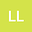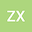G-CONTINUOUS MAPPINGS AND G-QUOTIENT MAPPINGS
••• liu li,
• ZHOU XIANGENG
liu li
Ningde Normal University

Corresponding Author:429378220@qq.com

Author ProfileZHOU XIANGENG
Ningde Normal University
Author Profile## Abstract

As a generalization of the usual convergence in topological spaces, a method $G$ on a set $X$ is a function $G: c_G(X)\to X$ defined on a subset $c_G(X)$ which is constituted by some sequences in $X$. In this paper, we mainly study the $G$-continuous mappings and the $G$-quotient mappings determined by $G$-methods and their connections with continuous mappings and quotient mappings in topological spaces. At the same time, we also discuss some properties of $G$-open mappings and $G$-closed mappings, and unify some results of several important convergence of sequences involving continuous mappings and quotient mappings.